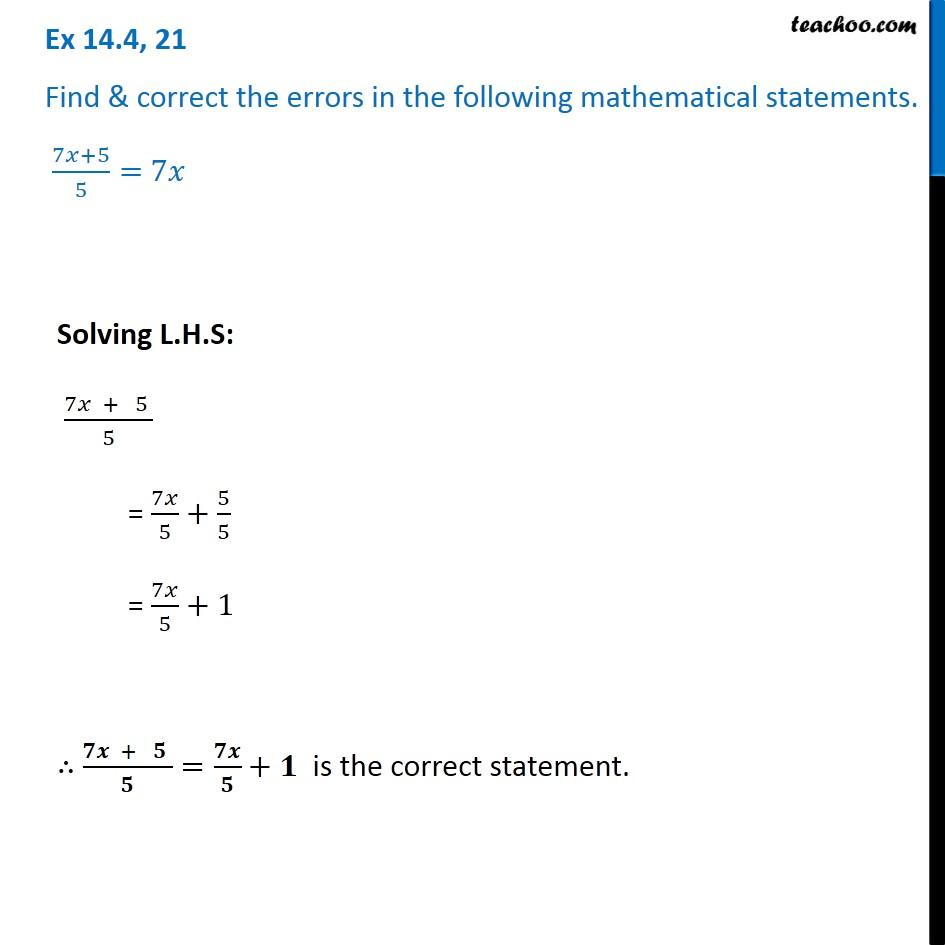Identify and correct errors in the sentences

Chapter 12 Class 8 Factorisation
Serial order wiseLearn in your speed, with individual attention - Teachoo Maths 1-on-1 Class

### Transcript

Question 21 Find & correct the errors in the following mathematical statements. (7𝑥+5)/5= 7𝑥 Solving L.H.S: (7𝑥 + 5 )/5 = 7𝑥/5+5/5 = 7𝑥/5+1 ∴ (𝟕𝒙 + 𝟓 )/𝟓=𝟕𝒙/𝟓+𝟏 is the correct statement.